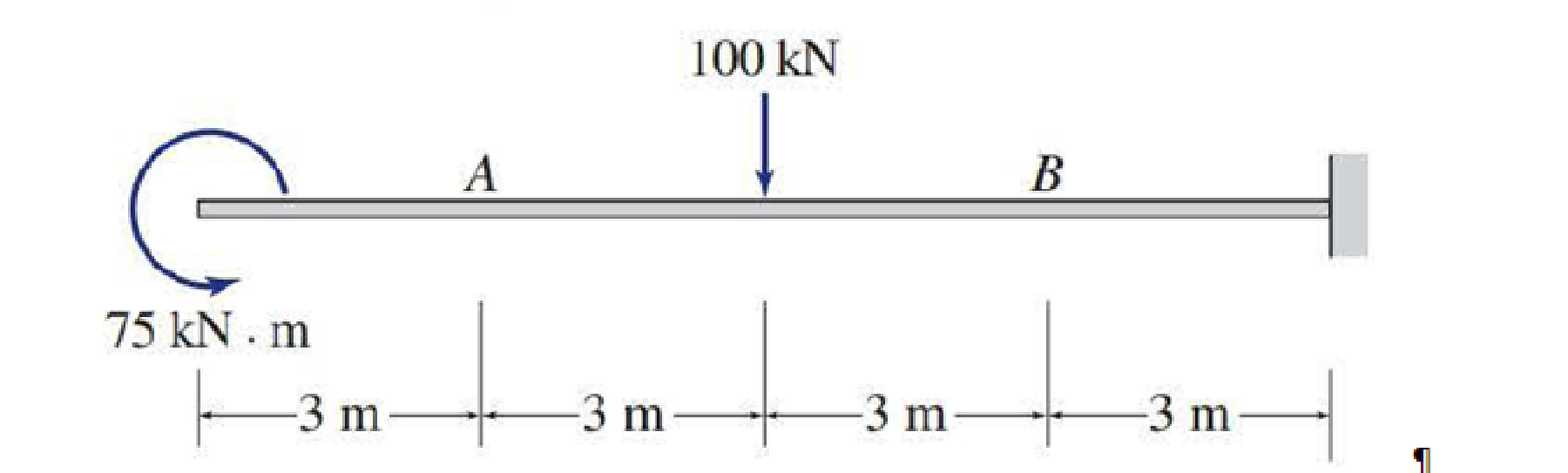# Determine the axial forces, shears, and bending moments at points A and B of the structure shown.

#### Solutions

Chapter
Section
Chapter 5, Problem 3P
Textbook Problem
545 views

## Determine the axial forces, shears, and bending moments at points A and B of the structure shown.To determine

Find the axial force, shear, and bending moment at points A and B of the beam.

### Explanation of Solution

Sign conversion:

Apply the sign convention for calculating the equations of equilibrium as below.

• For the horizontal forces equilibrium condition, take the force acting towards right side as positive (+) and the force acting towards left side as negative ().
• For the vertical forces equilibrium condition, take the upward force as positive (+) and downward force as negative ().
• For moment equilibrium condition, take the clockwise moment as negative and counter clockwise moment as positive.

Apply the following sign convention for calculating the axial forces, shear and bending moments.

• When the portion of the beam considered is left of the section, then the external force acting to the left are considered as positive.
• When the portion of the beam considered is right of the section, then the external force acting to the right are considered as positive.
• When the portion of the beam considered is left of the section, then the external force acting upward are considered as positive.
• When the portion of the beam considered is right of the section, then the external force acting downward are considered as positive.
• When the portion of the beam considered is left of the section, then the clockwise moments are considered as positive.
• When the portion of the beam considered is right of the section, then the counterclockwise moments are considered as positive.

Calculation:

Show the free-body diagram of the entire beam as in Figure 1.

Find the horizontal reaction at point D by resolving the horizontal equilibrium.

+Fx=0Dx=0Dx=0

Find the vertical reaction at point D by resolving the vertical equilibrium.

+Fy=0100+Dy=0Dy=100kN

Find the moment at point D by taking moment about the point D.

+MD=075+100(6)MD=075+600MD=0MD=675kN-m

Pass the sections aa and bb at points A and B respectively.

Show the sections aa and bb as in Figure 2.

Consider section aa:

Consider the left side of the section aa for calculation of internal forces.

Show the free-body diagram of the left side of the section aa as in Figure 3.

Find the axial force at point A by resolving the horizontal equilibrium.

+Fx=0QA=0QA=0

Find the shear at point A by resolving the vertical equilibrium

### Still sussing out bartleby?

Check out a sample textbook solution.

See a sample solution

#### The Solution to Your Study Problems

Bartleby provides explanations to thousands of textbook problems written by our experts, many with advanced degrees!

Get Started

Find more solutions based on key concepts
VocabularyState the meaning of the following terms: Orthographic Views _____ Isometric Drawing _____ Sectional ...

Engineering Fundamentals: An Introduction to Engineering (MindTap Course List)

What are the traditional 3 Vs of Big Data? Briefly, define each.

Database Systems: Design, Implementation, & Management

What is manufacturing?

Precision Machining Technology (MindTap Course List)

Virtual Machine Error You use virtual machines on your office computer so that you can run and test software in...

Enhanced Discovering Computers 2017 (Shelly Cashman Series) (MindTap Course List)

What steps are required to create an ODBC data source name?

Database Systems: Design, Implementation, & Management

What protects the molten weld metal from atmospheric contamination in GTAW welding?

Welding: Principles and Applications (MindTap Course List)

If your motherboard supports ECC DDR3 memory, can you substitute non-ECC DDR3 memory?

A+ Guide to Hardware (Standalone Book) (MindTap Course List)